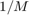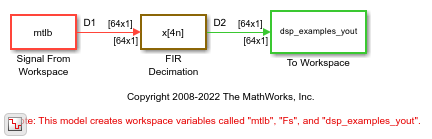# FIR Decimation Using Multirate Frame-Based Processing

In this example, the FIR Decimation block decimates the signal by decreasing the sample rate of the signal, while maintaining the frame size.

Open the model. The input to the FIR Decimation block is a single-channel input with a frame size of 64 and a frame period of 1 second. In the block dialog box, set the Decimation factor parameter to 4 and the Rate options parameter to `Allow multirate processing`.

Run the model. The block operates in the multirate frame-based processing mode. The input and output signals of the FIR Decimation block have the same frame size, but the sample rate of the output istimes the sample rate of the input, where M is the decimation factor. With a decimation factor of 4, the sample rate of the output signal is 1/4 times the sample rate of the input. The color coding in the model shows the sample time of the signal at both the input and output ports of the FIR Decimation block.## SupportGet trial now